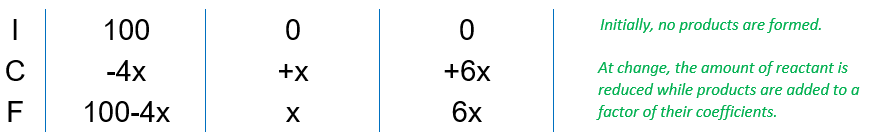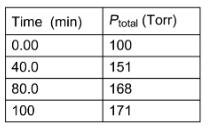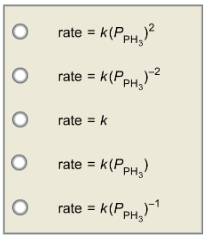# Problem: Phosphine, PH3(g), decomposes according to the equation 4PH3(g) → P4(g) + 6H2 (g) The kinetics of the decomposition of phosphine at 950 K was followed by measuring the total pressure in the system as a function of time. The data to the right were obtained in a run where the reaction chamber contained only pure phosphine at the start of the reaction. Choose the rate law that describes this reaction. Calculate the value of the rate constant and choose the correct units.k =

###### FREE Expert Solution

Part A.

We are asked to determine the Rate Law that describes the decomposition of Phosphine, given the temperature and the total system pressure as a function of time.

The chemical equation is:

4PH3(g)  →   P4(g) + 6H2(g)

Recall that the rate of reaction is the change in pressure (in this case) of a reactant for every change in timeSince we are given with the Total pressure of the system and we are only concerned with the reactant, we need to first identify the Pressure of PHfor every given time.

At t=0, there was only PH3, therefore:

${P}_{Total}={P}_{P{H}_{3}}=$100 torr

At t=40 min, we need to use the ICF chart to  determine the pressure of PH3.

4PH3(g)   →       P4(g)         +       6H2(g)At t=40 min, total system pressure is 151 torr, therefore,

$100-4x+x+6x=151\phantom{\rule{0ex}{0ex}}100+3x=151\phantom{\rule{0ex}{0ex}}3x=151-100\phantom{\rule{0ex}{0ex}}\frac{\overline{)3}x}{\overline{)3}}=\frac{51}{3}$

x = 17

Therefore, pressure of PHat t=40 is:

At t=40 min, pressure of PH3 is 32 torr.

At t=80 min, total system pressure is 168 torr, therefore,

$100-4x+x+6x=168\phantom{\rule{0ex}{0ex}}100+3x=168\phantom{\rule{0ex}{0ex}}3x=168-100\phantom{\rule{0ex}{0ex}}\frac{\overline{)3}x}{\overline{)3}}=\frac{68}{3}$

x = 22.7

Therefore, pressure of PHat t=80 is:

At t=80 min, pressure of PH3 is 9.2 torr.

At t=100 min, total system pressure is 171 torr, therefore,

$100-4x+x+6x=171\phantom{\rule{0ex}{0ex}}100+3x=171\phantom{\rule{0ex}{0ex}}3x=171-100\phantom{\rule{0ex}{0ex}}\frac{\overline{)3}x}{\overline{)3}}=\frac{71}{3}$

x = 23.7

Therefore, pressure of PHat t=100 is:

At t=100 min, pressure of PH3 is 5.2 torr.

89% (50 ratings)###### Problem Details

Phosphine, PH3(g), decomposes according to the equation

4PH3(g) → P4(g) + 6H(g)The kinetics of the decomposition of phosphine at 950 K was followed by measuring the total pressure in the system as a function of time. The data to the right were obtained in a run where the reaction chamber contained only pure phosphine at the start of the reaction. Choose the rate law that describes this reaction.Calculate the value of the rate constant and choose the correct units.

k =

Frequently Asked Questions

What scientific concept do you need to know in order to solve this problem?

Our tutors have indicated that to solve this problem you will need to apply the Integrated Rate Law concept. You can view video lessons to learn Integrated Rate Law. Or if you need more Integrated Rate Law practice, you can also practice Integrated Rate Law practice problems.

What professor is this problem relevant for?

Based on our data, we think this problem is relevant for Professor TBA's class at UBC.